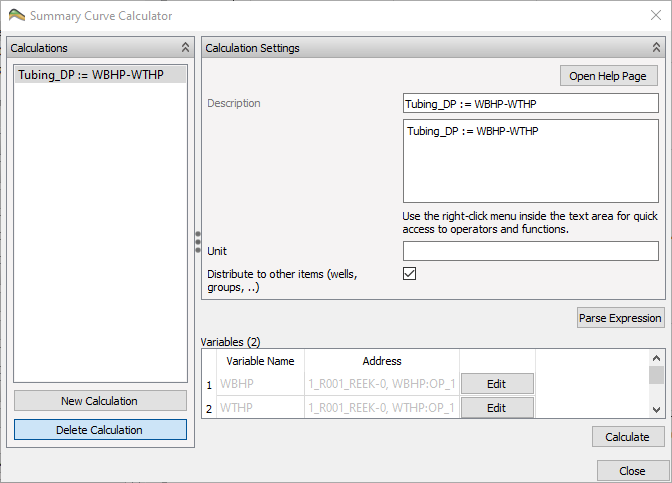# Calculator ExpressionsTwo similar calculators use expressions, summary curve calculator and grid cell calculator. These two variants share the text parsing for calculator expressions.

Grid Property Calculator

Summary Curve Calculator

ResInsight supports a subset of the features supported in the exprtk parsing library. Scripting features like for/while loops are not supported. The full documentation for the expression parser is available at C++ Mathematical Expression Toolkit Library

## Operators and Functions

Possible operations and functions are found by right-clicking in the expression window.

### Comment lines

Create a comment line by prefixing with // or ##

// Valid comment line 2
## Valid comment line 1


### Assignment Operators

OPERATORDEFINITION
:=Assignment
## Example
MY_VARIABLE := b + c


### Basic Operators

OPERATORDEFINITION
-Subtraction
*Multiplication
/Division
%Modulus
^Power
## Example
a := (b * c) + d / 10.5


### Conditionals

if-then-else statements can be used to assign values based on the individual values in a vector. The following case will assign 0.01 if the TRANX is below 0.01, else copy the original TRANX value.

## Example
NEW_TRANX := if((TRANX < 0.01), 0.01, TRANX)


### Scalar Functions

FUNCTIONDEFINITION
avgAverage
maxMaximum
minMinimum
sumSum
## Example: Use the accumulated sum for all PORV values to compute the normalized PORV
NORMALIZED_PORV := x/sum(PORV)


### Trigonometry Functions

FUNCTIONDEFINITION
cosCosine
coshHyperbolic cosine
cotCotangent
cscCosecant
secSecant
sinSine
sincSine cardinal
sinhHyperbolic sine
tanTangent
tanhHyperbolic tangent

### Vector Functions

FUNCTIONDEFINITION
absAbsolute value
ceilRounding up
floorRounding down
fracFractional portion of input
logNatural logarithm
log10Base 10 logarithm
powPower
roundRound x to the nearest integer
sgnSign of x, -1 where x < 0, +1 where x > 0, else zero
sqrtSquare root
truncInteger portion of input